# Filled contour plot in python

I’ve been making a chart that looks similar to this for a few different projects at work, so figured a quick blog post to show the notes of it would be useful.

So people often talk about setting a decision threshold to turn a predicted probability into a binary yes/no decision. E.g. do I do some process to this observation if the probability is 20%, 30%, 60%, etc. If you can identify the costs and benefits of making particular decisions, you can set a simple threshold to make that decision. For example, say you are sending adverts in the mail for a product. If the person buys the product, your company makes $50, and the advert only costs$1 to send. In this framework, if you have a predictive model for the probability the advert will be successful, then your decision threshold will look like this:

$50*probability -$1

So in this case you need the predicted probability to be above 2% to have an expected positive return on the investment of sending the advert. So if you have a probability of 10% for 2000 customers, you would expect to make 2000 * (50*0.1 - 1) = 8000. The probabilities you get from your predictive model can be thought of as in the long run averages. Any single advert may be a bust, but if your model is right and you send out a bunch, you should make this much money in the end. (If you have a vector of varying probabilities, in R code the estimated revenue will then look like prob <- runif(2000,0,0.1); pover <- prob > 0.02; sum( (50*prob - 1)*pover ).)

But many of the decisions I work with are not a single number in the benefits column. I am working with medical insurance claims data at HMS, and often determining models to audit those claims in some way. In this framework, it is more important to audit a high dollar claim than a lower dollar claim, even if the higher dollar value claim has a lower probability. So I have been making the subsequent filled contour plot I am going to show in the next section to illustrate this.

# python contour plot

The code snippet is small enough to just copy-paste entirely. First, I generate data over a regular grid to illustrate different claim amounts and then probabilities. Then I use np.meshgrid to get the data in the right shape for the contour plot. The revenue estimates are then simply the probability times the claims amount, minus some fixed (often labor to audit the claim) cost. After that is is just idiosyncratic matplotlib code to make a nice filled contour.

# Example of making a revenue contour plot
import matplotlib.pyplot as plt
from matplotlib.ticker import StrMethodFormatter
import numpy as np

n = 500 #how small grid cells are
prob = np.linspace(0,0.5,n)
dollar = np.linspace(0,10000,n)
#np.logspace(0,np.log10(10000),n) #if you want to do logged

# Generate grid
X, Y = np.meshgrid(prob, dollar)

# Example generating revenue
fixed = 200
Rev = (Y*X) - fixed

fig, ax = plt.subplots()
CS = ax.contourf(X, Y, Rev, cmap='RdPu')
clb = fig.colorbar(CS)
#clb.ax.set_xlabel('Revenue') #Abit too wide
clb.ax.set_title('dollar') #html does not like the dollar sign
ax.set_xlabel('Probability')
ax.set_ylabel('Claim Amount')
ax.yaxis.set_major_formatter(StrMethodFormatter('${x:,.0f}')) plt.title('Revenue Contours') plt.xticks(np.arange(0,0.6,0.1)) plt.yticks(np.arange(0,11000,1000)) plt.annotate('Revenue subtracts$200 of fixed labor costs',
(0,0), (0, -50),
xycoords='axes fraction',
textcoords='offset points', va='top')
#plt.savefig('RevContour.png',dpi=500,bbox_inches='tight')
plt.show()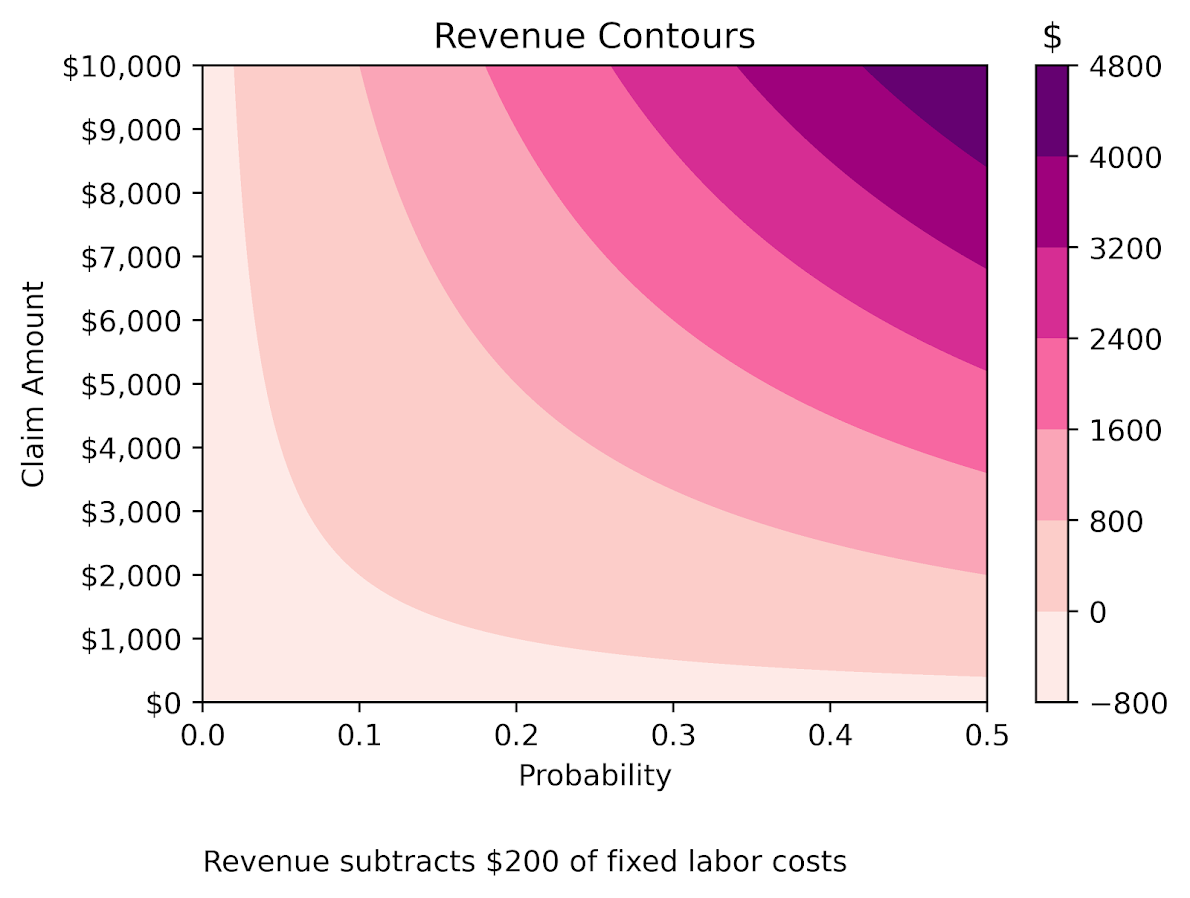The color bar does nice here out of the box. Next up in my personal learning will be how to manipulate color bars a bit more. Here I may want to either use a mask to not show negative expected returns, or a diverging color scheme (e.g. blue for negative returns).

# Making smoothed scatterplots in python

The other day I made a blog post on my notes on making scatterplots in matplotlib. One big chunk of why you want to make scatterplots though is if you are interested in a predictive relationship. Typically you want to look at the conditional value of the Y variable based on the X variable. Here are some example exploratory data analysis plots to accomplish that task in python.

I have posted the code to follow along on github here, in particular smooth.py has the functions of interest, and below I have various examples (that are saved in the Examples_Conditional.py file).

# Data Prep

First to get started, I am importing my libraries and loading up some of the data from my dissertation on crime in DC at street units. My functions are in the smooth set of code. Also I change the default matplotlib theme using smooth.change_theme(). Only difference from my prior posts is I don’t have gridlines by default here (they can be a bit busy).

#################################
import pandas as pd
import numpy as np
import matplotlib.pyplot as plt
import seaborn as sns
import statsmodels.api as sm
import os
import sys

mydir = r'D:\Dropbox\Dropbox\PublicCode_Git\Blog_Code\Python\Smooth'
data_loc = r'https://dl.dropbox.com/s/79ma3ldoup1bkw6/DC_CrimeData.csv?dl=0'
os.chdir(mydir)

#My functions
sys.path.append(mydir)
import smooth
smooth.change_theme()

#Dissertation dataset, can read from dropbox
#################################

# Binned Conditional Plots

The first set of examples, I bin the data and estimate the conditional means and standard deviations. So here in this example I estimate E[Y | X = 0], E[Y | X = 1], etc, where Y is the total number of part 1 crimes and x is the total number of alcohol licenses on the street unit (e.g. bars, liquor stores, or conv. stores that sell beer).

The function name is mean_spike, and you pass in at a minimum the dataframe, x variable, and y variable. I by default plot the spikes as +/- 2 standard deviations, but you can set it via the mult argument.

####################
#Example binning and making mean/std dev spike plots

smooth.mean_spike(DC_crime,'TotalLic','TotalCrime')

mean_lic = smooth.mean_spike(DC_crime,'TotalLic','TotalCrime',
plot=False,ret_data=True)
####################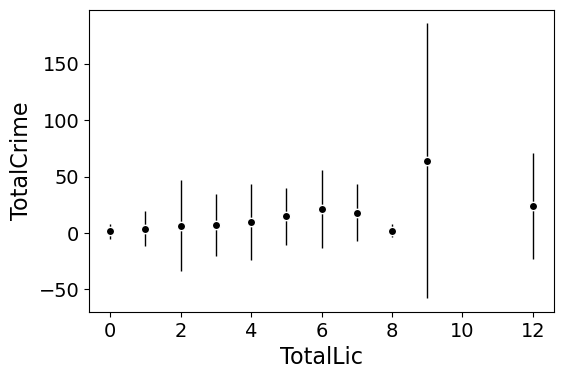This example works out because licenses are just whole numbers, so it can be binned. You can pass in any X variable that can be binned in the end. So you could pass in a string for the X variable. If you don’t like the resulting format of the plot though, you can just pass plot=False,ret_data=True for arguments, and you get the aggregated data that I use to build the plots in the end.

mean_lic = smooth.mean_spike(DC_crime,'TotalLic','TotalCrime',
plot=False,ret_data=True)

Another example I am frequently interested in is proportions and confidence intervals. Here it uses exact binomial confidence intervals at the 99% confidence level. Here I clip the burglary data to 0/1 values and then estimate proportions.

####################
#Example with proportion confidence interval spike plots

DC_crime['BurgClip'] = DC_crime['OffN3'].clip(0,1)
smooth.prop_spike(DC_crime,'TotalLic','BurgClip')

####################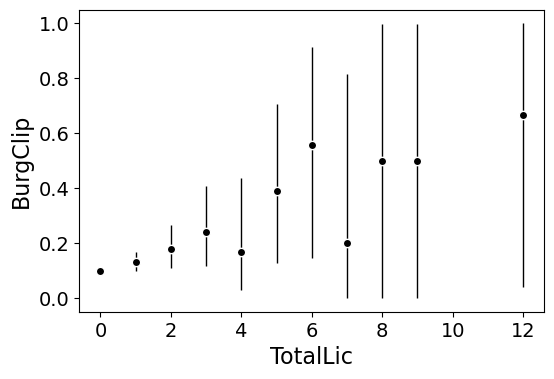A few things to note about this is I clip out bins with only 1 observation in them for both of these plots. I also do not have an argument to save the plot. This is because I typically only use these for exploratory data analysis, it is pretty rare I use these plots in a final presentation or paper.

I will need to update these in the future to jitter the data slightly to be able to superimpose the original data observations. The next plots are a bit easier to show that though.

# Restricted Cubic Spline Plots

Binning like I did prior works out well when you have only a few bins of data. If you have continuous inputs though it is tougher. In that case, typically what I want to do is estimate a functional relationship in a regression equation, e.g. Y ~ f(x), where f(x) is pretty flexible to identify potential non-linear relationships.

Many analysts are taught the loess linear smoother for this. But I do not like loess very much, it is often both locally too wiggly and globally too smooth in my experience, and the weighting function has no really good default.

Another popular choice is to use generalized additive model smoothers. My experience with these (in R) is better than loess, but they IMO tend to be too aggressive, and identify overly complicated functions by default.

My favorite approach to this is actually then from Frank Harrell’s regression modeling strategies. Just pick a regular set of restricted cubic splines along your data. It is arbitrary where to set the knot locations for the splines, but my experience is they are very robust (so chaning the knot locations only tends to change the estimated function form by a tiny bit).

I have class notes on restricted cubic splines I think are a nice introduction. First, I am going to make the same dataset from my class notes, the US violent crime rate from 85 through 2010.

years = pd.Series(list(range(26)))
vcr = [1881.3,
1995.2,
2036.1,
2217.6,
2299.9,
2383.6,
2318.2,
2163.7,
2089.8,
1860.9,
1557.8,
1344.2,
1268.4,
1167.4,
1062.6,
945.2,
927.5,
789.6,
734.1,
687.4,
673.1,
637.9,
613.8,
580.3,
551.8,
593.1]

yr_df = pd.DataFrame(zip(years,years+1985,vcr), columns=['y1','years','vcr'])

I have a function that allows you to append the spline basis to a dataframe. If you don’t pass in a data argument, in returns a dataframe of the basis functions.

#Can append rcs basis to dataframe
kn = [3.0,7.0,12.0,21.0]
smooth.rcs(years,knots=kn,stub='S',data=yr_df)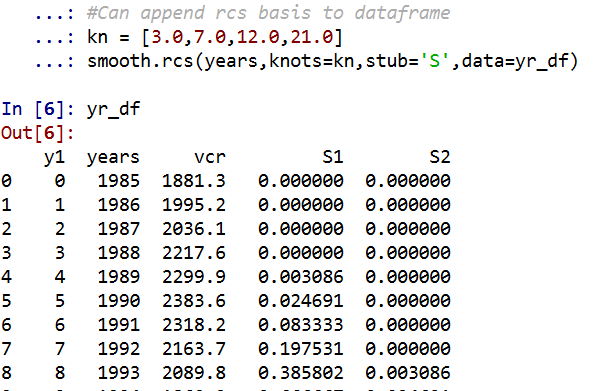I also have in the code set Harrell’s suggested knot locations for the data. This ranges from 3 to 7 knots (it will through an error if you pass a number not in that range). This here suggests the locations [1.25, 8.75, 16.25, 23.75].

#If you want to use Harrell's rules to suggest knot locations
smooth.sug_knots(years,4)

Note if you have integer data here these rules don’t work out so well (can have redundant suggested knot locations). So Harell’s defaults don’t work with my alcohol license data. But it is one of the reasons I like these though, I just pick regular locations along the X data and they tend to work well. So here is a regression plot passing in those knot locations kn = [3.0,7.0,12.0,21.0] I defined a few paragraphs ago, and the plot does a few vertical guides to show the knot locations.

#RCS plot
smooth.plot_rcs(yr_df,'y1','vcr',knots=kn)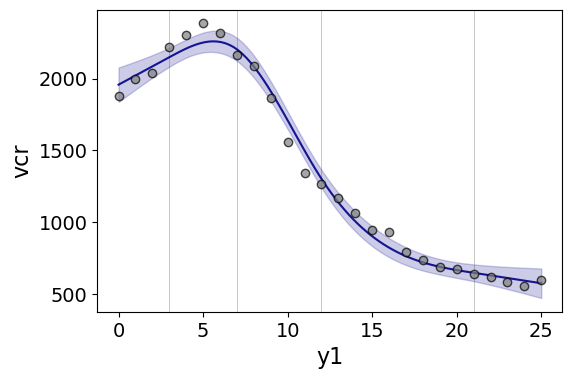Note that the error bands in the plot are confidence intervals around the mean, not prediction intervals. One of the nice things though about this under the hood, I used statsmodels glm interface, so if you want you can change the underlying link function to Poisson (I am going back to my DC crime data here), you just pass it in the fam argument:

#Can pass in a family argument for logit/Poisson models
smooth.plot_rcs(DC_crime,'TotalLic','TotalCrime', knots=[3,7,10,15],
fam=sm.families.Poisson(), marker_size=12)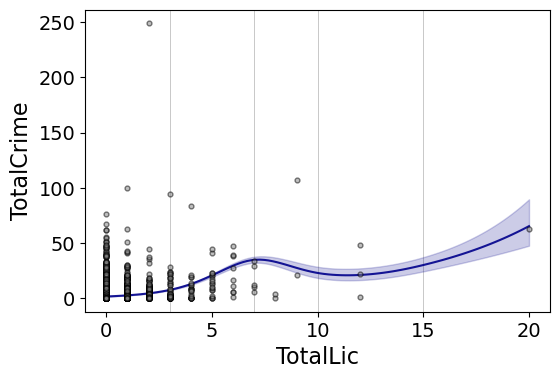This is a really great example for the utility of splines. I will show later, but a linear Poisson model for the alcohol license effect extrapolates very poorly and ends up being explosive. Here though the larger values the conditional effect fits right into the observed data. (And I swear I did not fiddle with the knot locations, there are just what I picked out offhand to spread them out on the X axis.)

And if you want to do a logistic regression:

smooth.plot_rcs(DC_crime,'TotalLic','BurgClip', knots=[3,7,10,15],
fam=sm.families.Binomial(),marker_alpha=0)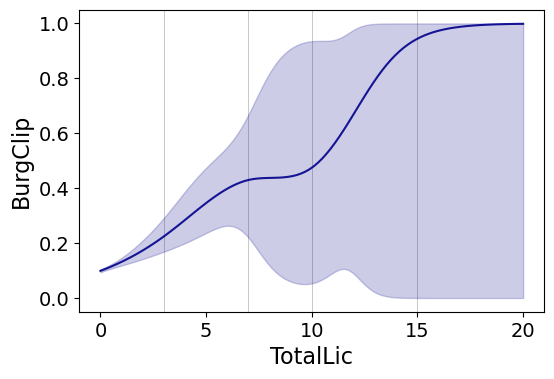I’m not sure how to do this in a way you can get prediction intervals (I know how to do it for Gaussian models, but not for the other glm families, prediction intervals probably don’t make sense for binomial data anyway). But one thing I could expand on in the future is to do quantile regression instead of glm models.

# Smooth Plots by Group

Sometimes you want to do the smoothed regression plots with interactions per groups. I have two helper functions to do this. One is group_rcs_plot. Here I use the good old iris data to illustrate, which I will explain why in a second.

#Superimposing rcs on the same plot
smooth.group_rcs_plot(iris,'sepal_length','sepal_width',
'species',colors=None,num_knots=3)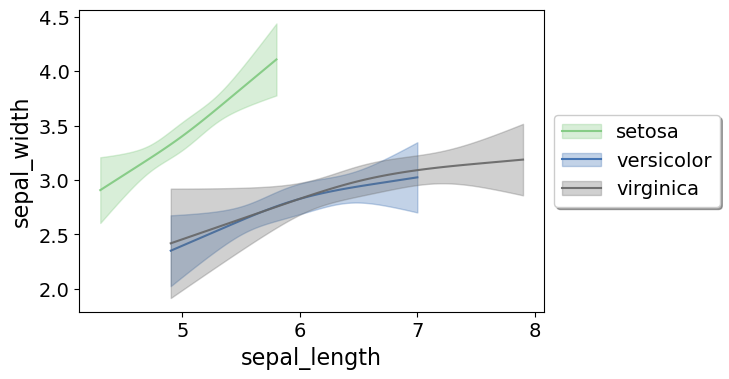If you pass in the num_knots argument, the knot locations are different for each subgroup of data (which I like as a default). If you pass in the knots argument and the locations, they are the same though for each subgroup.

Note that the way I estimate the models here I estimate three different models on the subsetted data frame, I do not estimate a stacked model with group interactions. So the error bands will be a bit wider than estimating the stacked model.

Sometimes superimposing many different groups is tough to visualize. So then a good option is to make a set of small multiple plots. To help with this, I’ve made a function loc_error, to pipe into seaborn’s small multiple set up:

#Small multiple example
g = sns.FacetGrid(iris, col='species',col_wrap=2)
g.map_dataframe(smooth.loc_error, x='sepal_length', y='sepal_width', num_knots=3)
g.set_axis_labels("Sepal Length", "Sepal Width")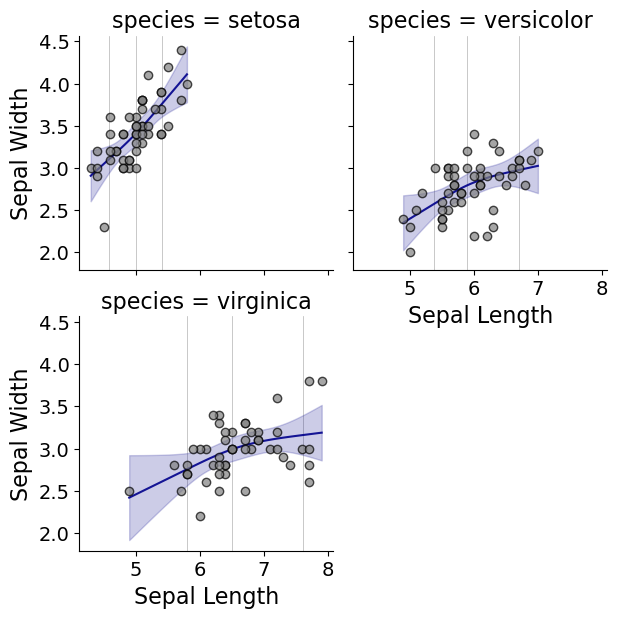And here you can see that the not locations are different for each subset, and this plot by default includes the original observations.

# Using the Formula Interface for Plots

Finally, I’ve been experimenting a bit with using the input in a formula interface, more similar to the way ggplot in R allows you to do this. So this is a new function, plot_form, and here is an example Poisson linear model:

smooth.plot_form(data=DC_crime,x='TotalLic',y='TotalCrime',
form='TotalCrime ~ TotalLic',
fam=sm.families.Poisson(), marker_size=12)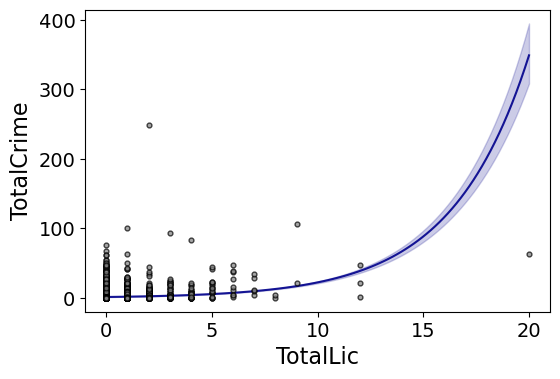You can see the explosive effect I talked about, which is common for Poisson/negative binomial models.

Here with the formula interface you can do other things, such as a polynomial regression:

#Can do polynomial terms
smooth.plot_form(data=DC_crime,x='TotalLic',y='TotalCrime',
form='TotalCrime ~ TotalLic + TotalLic**2 + TotalLic**3',
fam=sm.families.Poisson(), marker_size=12)

Which here ends up being almost indistinguishable from the linear terms. You can do other smoothers that are available in the patsy library as well, here are bsplines:

#Can do other smoothers
smooth.plot_form(data=DC_crime,x='TotalLic',y='TotalCrime',
form='TotalCrime ~ bs(TotalLic,df=4,degree=3)',
fam=sm.families.Poisson(), marker_size=12)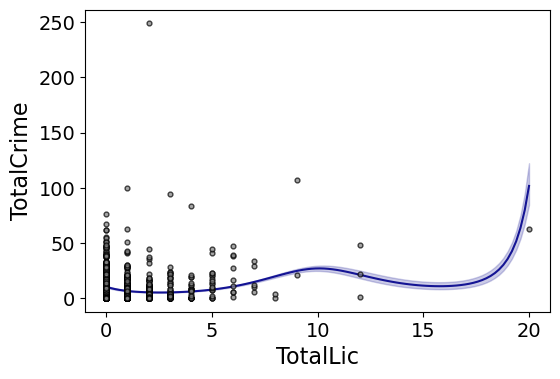I don’t really have a good reason to prefer restricted cubic splines to bsplines, I am just more familiar with restricted cubic splines (and this plot does not illustrate the knot locations that were by default chosen, although you could pass in knot locations to the bs function).

You can also do other transformations of the x variable. So here if you take the square root of the total number of licenses helps with the explosive effect somewhat:

#Can do transforms of the X variable
smooth.plot_form(data=DC_crime,x='TotalLic',y='TotalCrime',
form='TotalCrime ~ np.sqrt(TotalLic)',
fam=sm.families.Poisson(), marker_size=12)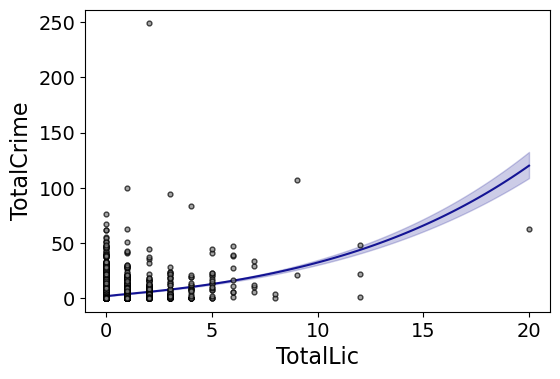In the prior blog post about explosive Poisson models I also showed a broken stick type model if you wanted to log the x variable but it has zero values.

#Can do multiple transforms of the X variable
smooth.plot_form(data=DC_crime,x='TotalLic',y='TotalCrime',
form='TotalCrime ~ np.log(TotalLic.clip(1)) + I(TotalLic==0)',
fam=sm.families.Poisson(), marker_size=12)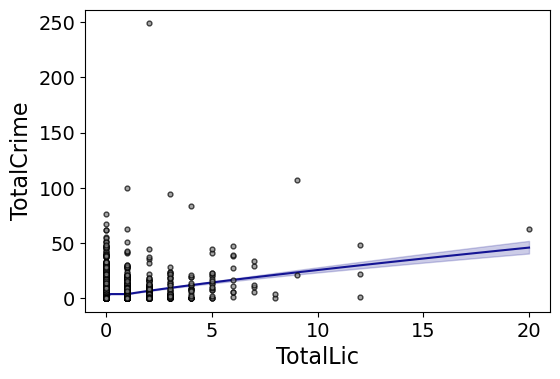Technically this “works” if you transform the Y variable as well, but the resulting plot is misleading, and the prediction interval is for the transformed variable. E.g. if you pass a formula 'np.log(TotalCrime+1) ~ TotalLic', you would need to exponentiate the the predictions and subtract 1 to get back to the original scale (and then the line won’t be the mean anymore, but the confidence intervals are OK).

I will need to see if I can figure out patsy and sympy to be able to do the inverse transformation to even do that. That type of transform to the y variable directly probably only makes sense for linear models, and then I would also maybe need to do a Duan type smearing estimate to get the mean effect right.

# Notes on making scatterplots in matplotlib and seaborn

Many of my programming tips, like my notes for making Leaflet maps in R or margins plots in Stata, I’ve just accumulated doing projects over the years. My current workplace is a python shop though, so I am figuring it out all over for some of these things in python. I made some ugly scatterplots for a presentation the other day, and figured it would be time to spend alittle time making some notes on making them a bit nicer.

For prior python graphing post examples, I have:

For this post, I am going to use the same data I illustrated with SPSS previously, a set of crime rates in Appalachian counties. Here you can download the dataset and the python script to follow along.

# Making scatterplots using matplotlib

So first for the upfront junk, I load my libraries, change my directory, update my plot theme, and then load my data into a dataframe crime_dat. I technically do not use numpy in this script, but soon as I take it out I’m guaranteed to need to use np. for something!

################################################################
import pandas as pd
import numpy as np
import os
import matplotlib
import matplotlib.pyplot as plt
import seaborn as sns

my_dir = r'C:\Users\andre\OneDrive\Desktop\big_scatter'
os.chdir(my_dir)

andy_theme = {'axes.grid': True,
'grid.linestyle': '--',
'legend.framealpha': 1,
'legend.facecolor': 'white',
'legend.fontsize': 14,
'legend.title_fontsize': 16,
'xtick.labelsize': 14,
'ytick.labelsize': 14,
'axes.labelsize': 16,
'axes.titlesize': 20,
'figure.dpi': 100}

matplotlib.rcParams.update(andy_theme)
################################################################

First, lets start from the base scatterplot. After defining my figure and axis objects, I add on the ax.scatter by pointing the x and y’s to my pandas dataframe columns, here Burglary and Robbery rates per 100k. You could also instead of starting from the matplotlib objects start from the pandas dataframe methods (as I did in my prior histogram post). I don’t have a good reason for using one or the other.

Then I set the axis grid lines to be below my points (is there a way to set this as a default?), and then I set my X and Y axis labels to be nicer than the default names.

################################################################
#Default scatterplot
fig, ax = plt.subplots(figsize=(6,4))
ax.scatter(crime_dat['burg_rate'], crime_dat['rob_rate'])
ax.set_axisbelow(True)
ax.set_xlabel('Burglary Rate per 100,000')
ax.set_ylabel('Robbery Rate per 100,000')
plt.savefig('Scatter01.png', dpi=500, bbox_inches='tight')
plt.show()
################################################################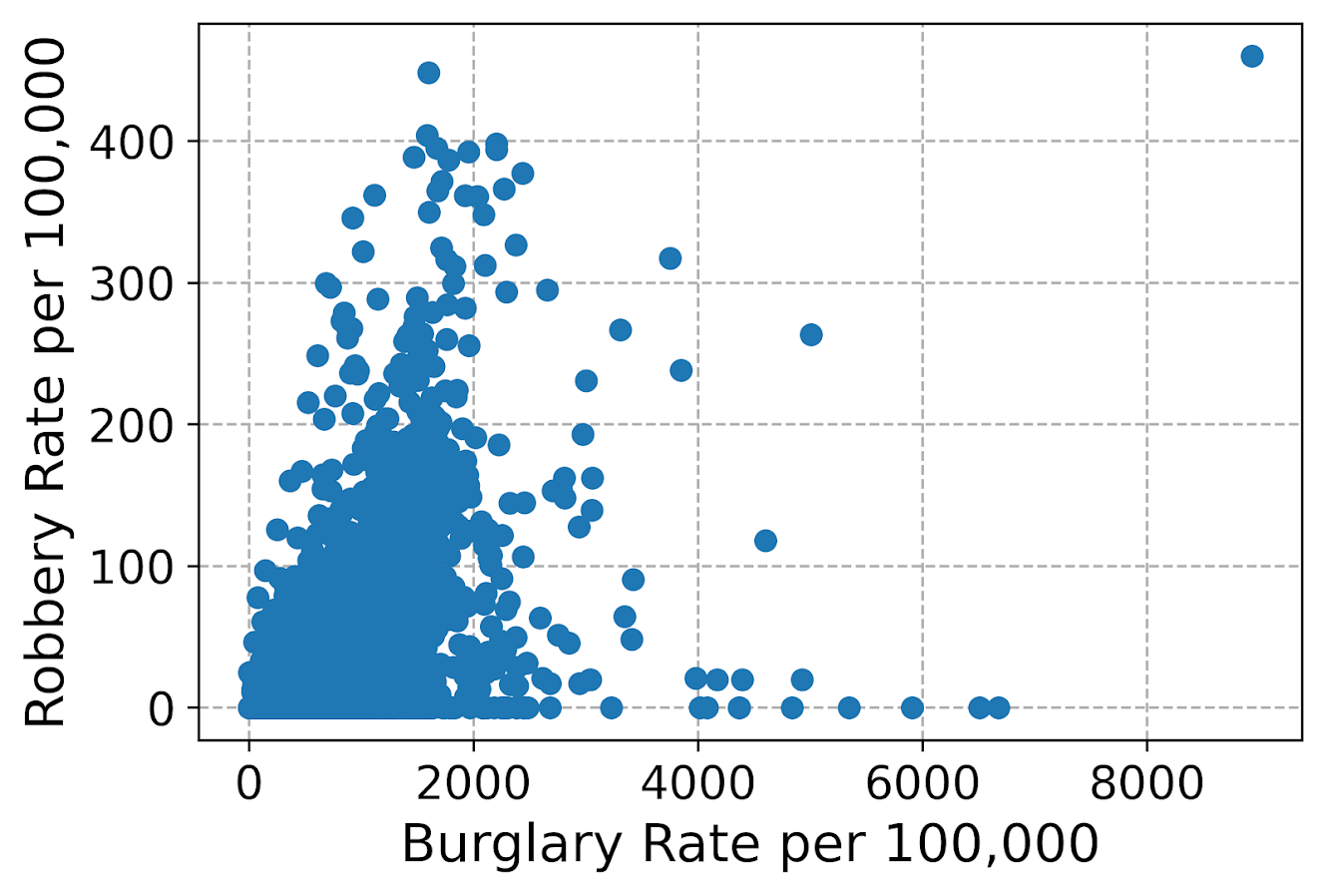You can see here the default point markers, just solid blue filled circles with no outline, when you get a very dense scatterplot just looks like a solid blob. I think a better default for scatterplots is to plot points with an outline. Here I also make the interior fill slightly transparent. All of this action is going on in the ax.scatter call, all of the other lines are the same.

################################################################
#Making points have an outline and interior fill
fig, ax = plt.subplots(figsize=(6,4))
ax.scatter(crime_dat['burg_rate'], crime_dat['rob_rate'],
c='grey', edgecolor='k', alpha=0.5)
ax.set_axisbelow(True)
ax.set_xlabel('Burglary Rate per 100,000')
ax.set_ylabel('Robbery Rate per 100,000')
plt.savefig('Scatter02.png', dpi=500, bbox_inches='tight')
plt.show()
################################################################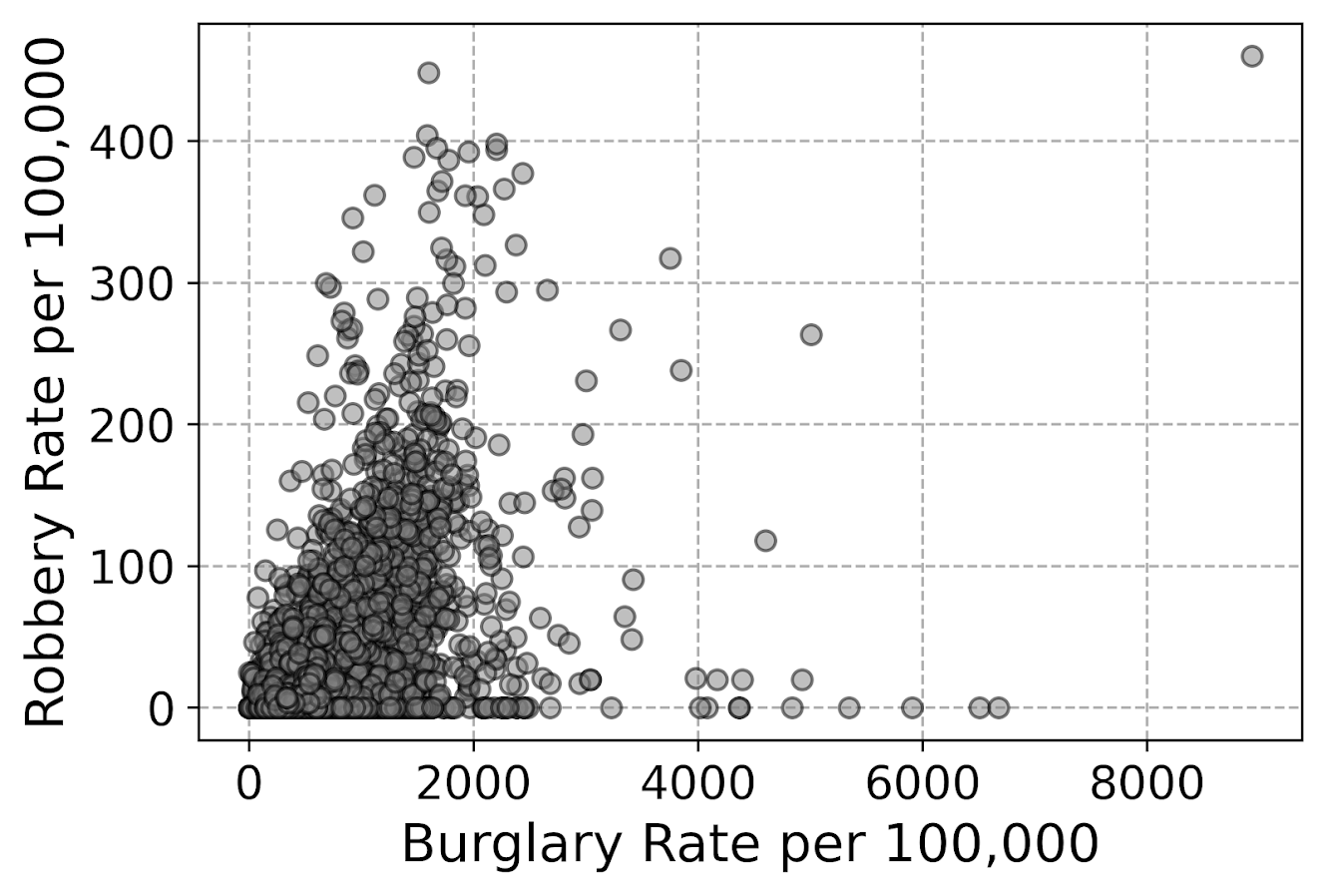So that is better, but we still have quite a bit of overplotting going on. Another quick trick is to make the points smaller and up the transparency by setting alpha to a lower value. This allows you to further visualize the density, but then makes it a bit harder to see individual points – if you started from here you might miss that outlier in the upper right.

Note I don’t set the edgecolor here, but if you want to make the edges semitransparent as well you could do edgecolor=(0.0, 0.0, 0.0, 0.5), where the last number of is the alpha transparency tuner.

################################################################
#Making the points small and semi-transparent
fig, ax = plt.subplots(figsize=(6,4))
ax.scatter(crime_dat['burg_rate'], crime_dat['rob_rate'], c='k',
alpha=0.1, s=4)
ax.set_axisbelow(True)
ax.set_xlabel('Burglary Rate per 100,000')
ax.set_ylabel('Robbery Rate per 100,000')
plt.savefig('Scatter03.png', dpi=500, bbox_inches='tight')
plt.show()
################################################################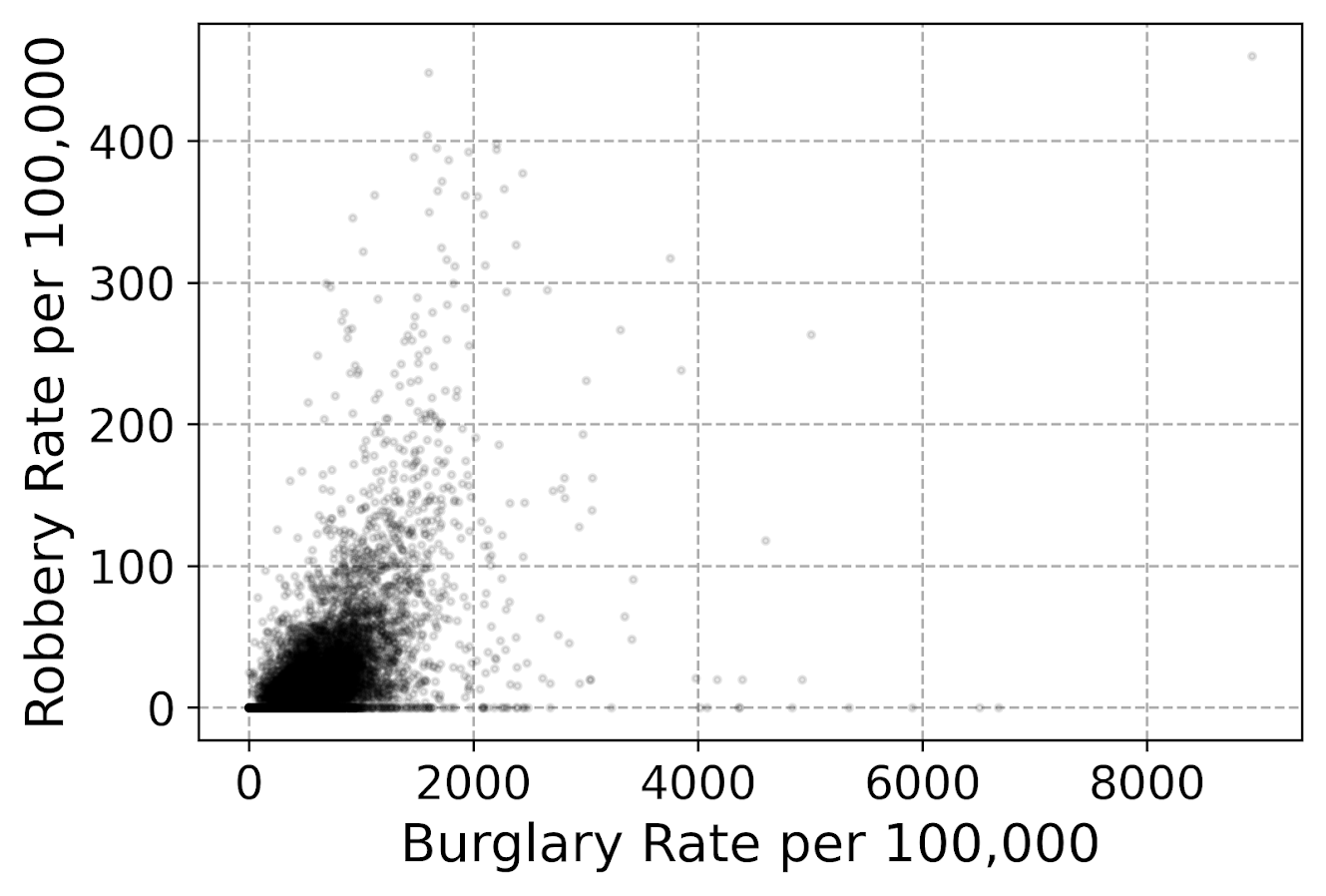This dataset has around 7.5k rows in it. For most datasets of anymore than a hundred points, you often have severe overplotting like you do here. One way to solve that problem is to bin observations, and then make a graph showing the counts within the bins. Matplotlib has a very nice hexbin method for doing this, which is easier to show than explain.

################################################################
#Making a hexbin plot
fig, ax = plt.subplots(figsize=(6,4))
hb = ax.hexbin(crime_dat['burg_rate'], crime_dat['rob_rate'],
gridsize=20, edgecolors='grey',
cmap='inferno', mincnt=1)
ax.set_axisbelow(True)
ax.set_xlabel('Burglary Rate per 100,000')
ax.set_ylabel('Robbery Rate per 100,000')
cb = fig.colorbar(hb, ax=ax)
plt.savefig('Scatter04.png', dpi=500, bbox_inches='tight')
plt.show()
################################################################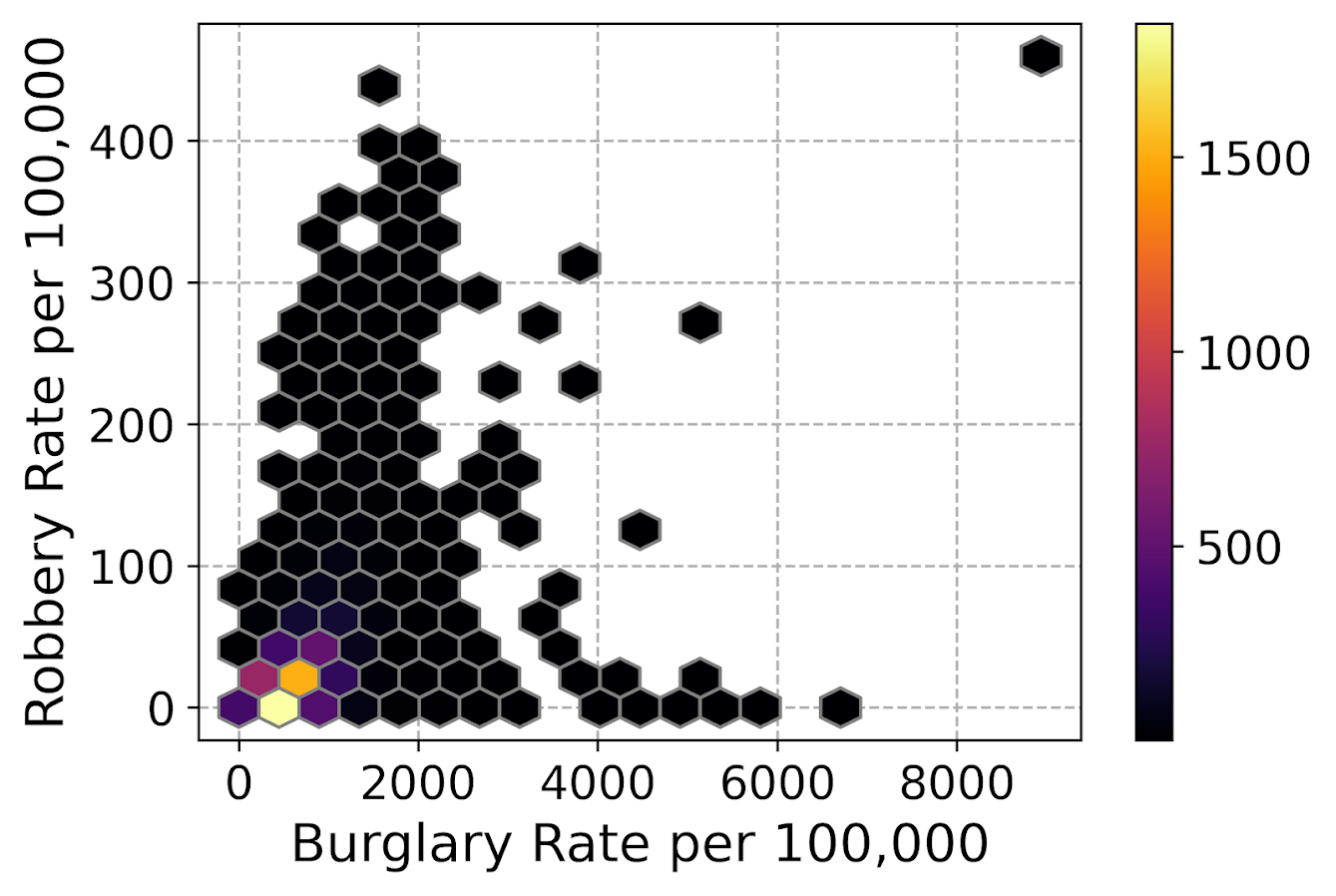So for the hexbins I like using the mincnt=1 option, as it clearly shows areas with no points, but then you can still spot the outliers fairly easy. (Using white for the edge colors looks nice as well.)

You may be asking, what is up with that outlier in the top right? It ends up being Letcher county in Kentucky in 1983, which had a UCR population estimate of only 1522, but had a total of 136 burglaries and 7 robberies. This could technically be correct (only some local one cop town reported, and that town does not cover the whole county), but I’m wondering if this is a UCR reporting snafu.

It is also a good use case for funnel charts. I debated on making some notes here about putting in text labels, but will hold off for now. You can add in text by using ax.annotate fairly easy by hand, but it is hard to automate text label positions. It is maybe easier to make interactive graphs and have a tooltip, but that will need to be another blog post as well.

# Making scatterplots using seaborn

The further examples I show are using the seaborn library, imported earlier as sns. I like using seaborn to make small multiple plots, but it also has a very nice 2d kernel density contour plot method I am showing off.

Note this does something fundamentally different than the prior hexbin chart, it creates a density estimate. Here it looks pretty but creates a density estimate in areas that are not possible, negative crime rates. (There are ways to prevent this, such as estimating the KDE on a transformed scale and retransforming back, or reflecting the density back inside the plot would probably make more sense here, ala edge weighting in spatial statistics.)

Here the only other things to note are used filled contours instead of just the lines, I also drop the lowest shaded area (I wish I could just drop areas of zero density, note dropping the lowest area drops my outlier in the top right). Also I have a tough go of using the default bandwidth estimators, so I input my own.

################################################################
#Making a contour plot using seaborn
g = sns.kdeplot(crime_dat['burg_rate'], crime_dat['rob_rate'],
g.set_axisbelow(True)
g.set_xlabel('Burglary Rate per 100,000')
g.set_ylabel('Robbery Rate per 100,000')
plt.savefig('Scatter05.png', dpi=500, bbox_inches='tight')
plt.show()
################################################################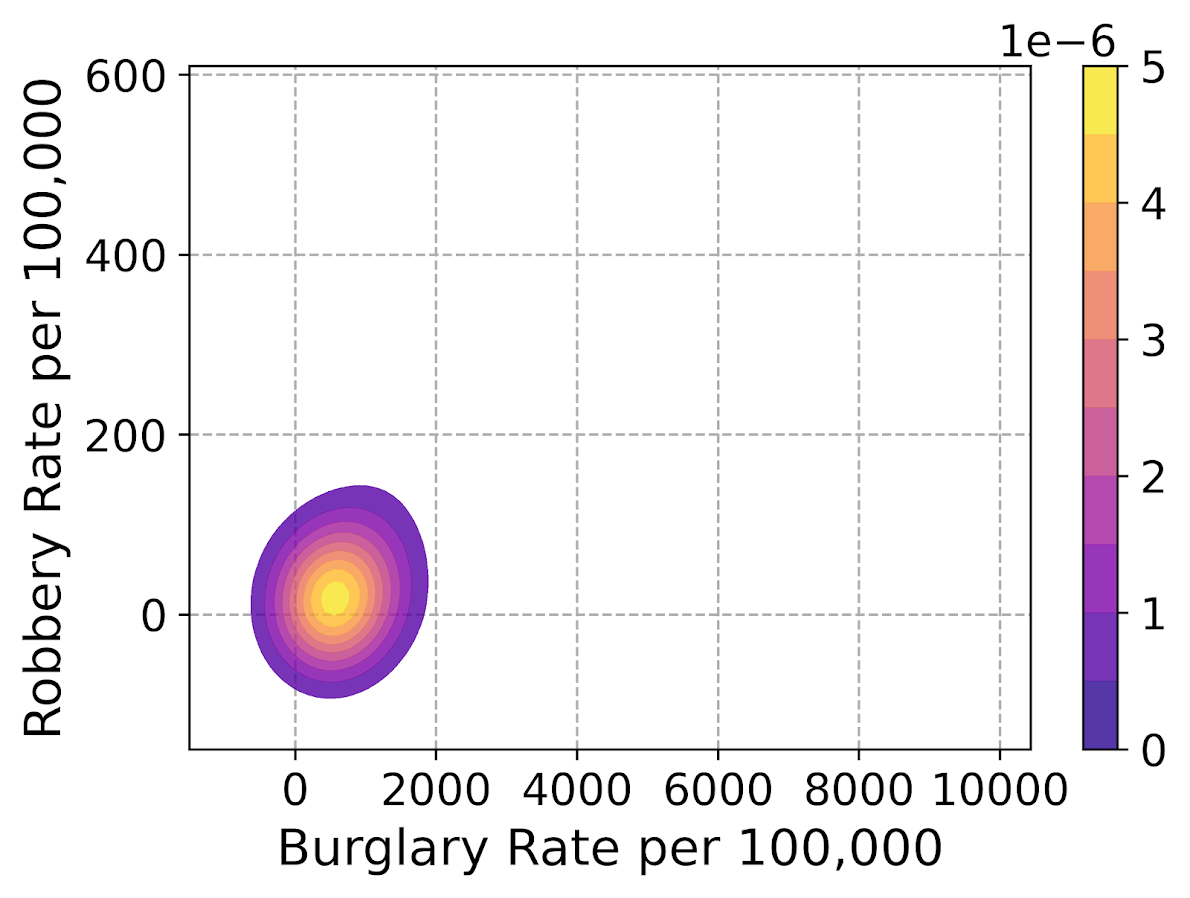So far I have not talked about the actual marker types. It is very difficult to visualize different markers in a scatterplot unless they are clearly separated. So although it works out OK for the Iris dataset because it is small N and the species are clearly separated, in real life datasets it tends to be much messier.

So I very rarely use multiple point types to symbolize different groups in a scatterplot, but prefer to use small multiple graphs. Here is an example of turning my original scatterplot, but differentiating between different county areas in the dataset. It is a pretty straightforward update using sns.FacetGrid to define the group, and then using g.map. (There is probably a smarter way to set the grid lines below the points for each subplot than the loop.)

################################################################
#Making a small multiple scatterplot using seaborn
g = sns.FacetGrid(data=crime_dat, col='subrgn',
col_wrap=2, despine=False, height=4)
g.map(plt.scatter, 'burg_rate', 'rob_rate', color='grey',
s=12, edgecolor='k', alpha=0.5)
g.set_titles("{col_name}")
for a in g.axes:
a.set_axisbelow(True)
g.set_xlabels('Burglary Rate per 100,000')
g.set_ylabels('Robbery Rate per 100,000')
plt.savefig('Scatter06.png', dpi=500, bbox_inches='tight')
plt.show()
################################################################And then finally I show an example of making a small multiple hexbin plot. It is alittle tricky, but this is an example in the seaborn docs of writing your own sub-plot function and passing that.

To make this work, you need to pass an extent for each subplot (so the hexagons are not expanded/shrunk in any particular subplot). You also need to pass a vmin/vmax argument, so the color scales are consistent for each subplot. Then finally to add in the color bar I just fiddled with adding an axes. (Again there is probably a smarter way to scoop up the plot coordinates for the last plot, but here I just experimented till it looked about right.)

################################################################
#Making a small multiple hexbin plot using seaborn

#https://stackoverflow.com/a/31385996/604456
def loc_hexbin(x, y, **kwargs):
kwargs.pop("color", None)
plt.hexbin(x, y, gridsize=20, edgecolor='grey',
cmap='inferno', mincnt=1,
vmin=1, vmax=700, **kwargs)

g = sns.FacetGrid(data=crime_dat, col='subrgn',
col_wrap=2, despine=False, height=4)
g.map(loc_hexbin, 'burg_rate', 'rob_rate',
edgecolors='grey', extent=[0, 9000, 0, 500])
g.set_titles("{col_name}")
for a in g.axes:
a.set_axisbelow(True)
#This goes x,y,width,height
cax = g.fig.add_axes([0.55, 0.09, 0.03, .384])
plt.colorbar(cax=cax, ax=g.axes)
g.set_xlabels('Burglary Rate per 100,000')
g.set_ylabels('Robbery Rate per 100,000')
plt.savefig('Scatter07.png', dpi=500, bbox_inches='tight')
plt.show()
################################################################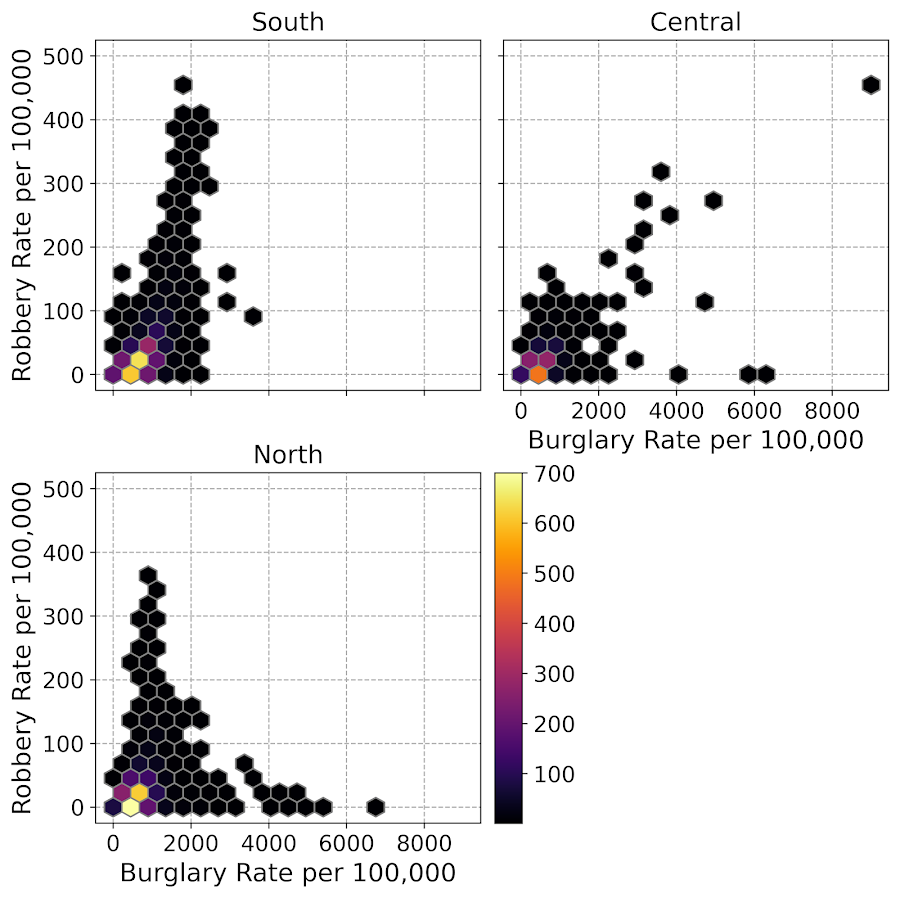Another common task with scatterplots is to visualize a smoother, e.g. E[Y|X] the expected mean of Y conditional on X, or you could do any other quantile, etc. That will have to be another post though, but for examples I have written about previously I have jittering 0/1 data, and visually weighted regression.

# Notes on matplotlib and seaborn charts (python)

My current workplace is a python shop. I actually didn’t use pandas/numpy for most of my prior academic projects, but I really like pandas for data manipulation now that I know it better. I’m using python objects (lists, dictionaries, sets) inside of data frames quite a bit to do some tricky data manipulations.

I do however really miss using ggplot to make graphs. So here are my notes on using python tools to make plots, specifically the matplotlib and seaborn libraries. Here is the data/code to follow along on your own.

# some set up

First I am going to redo the data analysis for predictive recidivism I did in a prior blog post. One change is that I noticed the default random forest implementation in sci-kit was prone to overfitting the data – so one simple regularization was to either limit depth of trees, or number of samples needed to split, or the total number of samples in a final leaf. (I noticed this when I developed a simulated example xgboost did well with the defaults, but random forests did not. It happened to be becauase xgboost defaults had a way smaller number of potential splits, when using similar defaults they were pretty much the same.)

Here I just up the minimum samples per leaf to 100.

#########################################################
#set up for libraries and data I need
import pandas as pd
import os
import numpy as np
from sklearn.ensemble import RandomForestClassifier
import matplotlib
import matplotlib.pyplot as plt
import seaborn as sns

my_dir = r'C:\Users\andre\Dropbox\Documents\BLOG\matplotlib_seaborn'
os.chdir(my_dir)

#Modelling recidivism using random forests, see below for background
#https://andrewpwheeler.com/2020/01/05/balancing-false-positives/

#Preparing the variables I want
recid_prep = recid[['Recid30','CompScore.1','CompScore.2','CompScore.3',
recid_prep['Male'] = 1*(recid['sex'] == "Male")
recid_prep['Fel'] = 1*(recid['c_charge_degree'] == "F")
recid_prep['Mis'] = 1*(recid['c_charge_degree'] == "M")
recid_prep['race'] = recid['race']

#Now generating train and test set
recid_prep['Train'] = np.random.binomial(1,0.75,len(recid_prep))
recid_train = recid_prep[recid_prep['Train'] == 1]
recid_test = recid_prep[recid_prep['Train'] == 0]

#Now estimating the model
ind_vars = ['CompScore.1','CompScore.2','CompScore.3',
dep_var = 'Recid30'
rf_mod = RandomForestClassifier(n_estimators=500, random_state=10, min_samples_leaf=100)
rf_mod.fit(X = recid_train[ind_vars], y = recid_train[dep_var])

#Now applying out of sample
pred_prob = rf_mod.predict_proba(recid_test[ind_vars] )
recid_test['prob'] = pred_prob[:,1]
#########################################################

# matplotlib themes

One thing you can do is easily update the base template for matplotlib. Here are example settings I typically use, in particular making the default font sizes much larger. I also like a using a drop shadow for legends – although many consider drop shadows for data chart-junky, they actually help distinguish the legend from the background plot (a trick I learned from cartographic maps).

#########################################################
#Settings for matplotlib base

andy_theme = {'axes.grid': True,
'grid.linestyle': '--',
'legend.framealpha': 1,
'legend.facecolor': 'white',
'legend.fontsize': 14,
'legend.title_fontsize': 16,
'xtick.labelsize': 14,
'ytick.labelsize': 14,
'axes.labelsize': 16,
'axes.titlesize': 20,
'figure.dpi': 100}

print( matplotlib.rcParams )
#matplotlib.rcParams.update(andy_theme)

#print(plt.style.available)
#plt.style.use('classic')
#########################################################

I have it commented out here, but once you define your dictionary of particular style changes, then you can just run matplotlib.rcParams.update(your_dictionary) to update the base plots. You can also see the ton of options by printing matplotlib.rcParams, and there are a few different styles already available to view as well.

# creating a lift-calibration line plot

Now I am going to create a plot that I have seen several names used for – I am going to call it a calibration lift-plot. Calibration is basically “if my model predicts something will happen 5% of the time, does it actually happen 5% of the time”. I used to always do calibration charts where I binned the data, and put the predicted on the X axis, and observed on the Y (see this example). But data-robot has an alternative plot, where you superimpose those two lines that has been growing on me.

#########################################################
#Creating a calibration lift-plot for entire test set

bin_n = 30
recid_test['Bin'] = pd.qcut(recid_test['prob'], bin_n, range(bin_n) ).astype(int) + 1
recid_test['Count'] = 1

agg_bins = recid_test.groupby('Bin', as_index=False)['Recid30','prob','Count'].sum()
agg_bins['Predicted'] = agg_bins['prob']/agg_bins['Count']
agg_bins['Actual'] = agg_bins['Recid30']/agg_bins['Count']

#Now can make a nice matplotlib plot
fig, ax = plt.subplots(figsize=(6,4))
ax.plot(agg_bins['Bin'], agg_bins['Predicted'], marker='+', label='Predicted')
ax.plot(agg_bins['Bin'], agg_bins['Actual'], marker='o', markeredgecolor='w', label='Actual')
ax.set_ylabel('Probability')
ax.legend(loc='upper left')
plt.savefig('Default_mpl.png', dpi=500, bbox_inches='tight')
plt.show()
#########################################################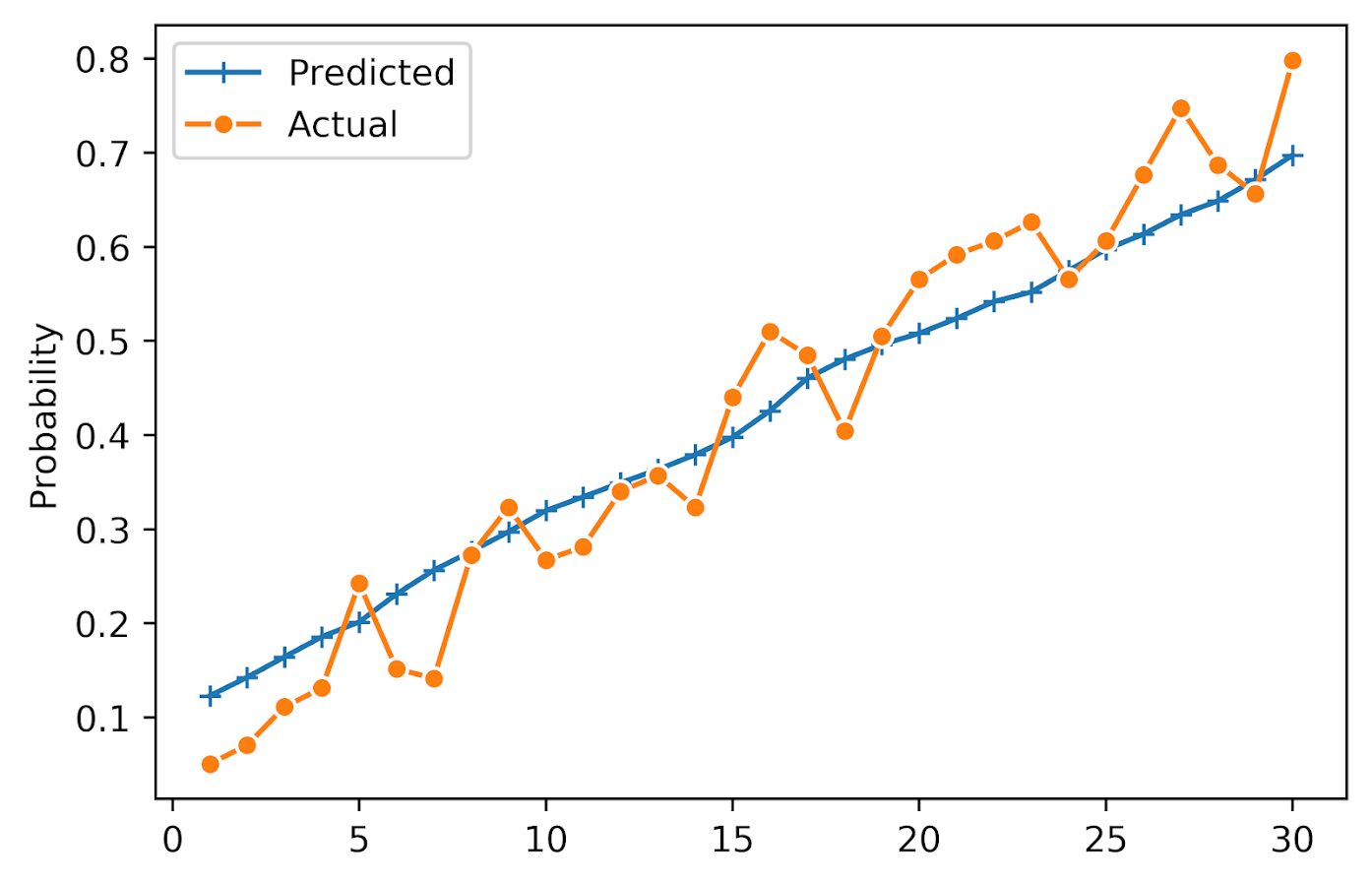You can see that the model is fairly well calibrated in the test set, and that the predictions range from around 10% to 75%. It is noisy and snakes high and low, but that is expected as we don’t have a real giant test sample here (around a total of 100 observations per bin).

So this is the default matplotlib style. Here is the slight update using my above specific theme.

matplotlib.rcParams.update(andy_theme)
fig, ax = plt.subplots(figsize=(6,4))
ax.plot(agg_bins['Bin'], agg_bins['Predicted'], marker='+', label='Predicted')
ax.plot(agg_bins['Bin'], agg_bins['Actual'], marker='o', markeredgecolor='w', label='Actual')
ax.set_ylabel('Probability')
ax.legend(loc='upper left')
plt.savefig('Mytheme_mpl.png', dpi=500, bbox_inches='tight')
plt.show()Not too different from the default, but I only have to call matplotlib.rcParams.update(andy_theme) one time and it will apply it to all my charts. So I don’t have to continually set the legend shadow, grid lines, etc.

# making a lineplot in seaborn

matplotlib is basically like base graphics in R, where if you want to superimpose a bunch of stuff you make the base plot and then add in lines() or points() etc. on top of the base. This is ok for only a few items, but if you have your data in long format, where a certain category distinguishes groups in the data, it is not very convenient.

The seaborn library provides some functions to get closer to the ggplot idea of mapping aesthetics using long data, so here is the same lineplot example. seaborn builds stuff on top of matplotlib, so it inherits the style I defined earlier. In this code snippet, first I melt the agg_bins data to long format. Then it is a similarish plot call to draw the graph.

#########################################################
#Now making the same chart in seaborn
#Easier to melt to wide data

agg_long = pd.melt(agg_bins, id_vars=['Bin'], value_vars=['Predicted','Actual'], var_name='Type', value_name='Probability')

plt.figure(figsize=(6,4))
sns.lineplot(x='Bin', y='Probability', hue='Type', style='Type', data=agg_long, dashes=False,
markers=True, markeredgecolor='w')
plt.xlabel(None)
plt.savefig('sns_lift.png', dpi=500, bbox_inches='tight')
#########################################################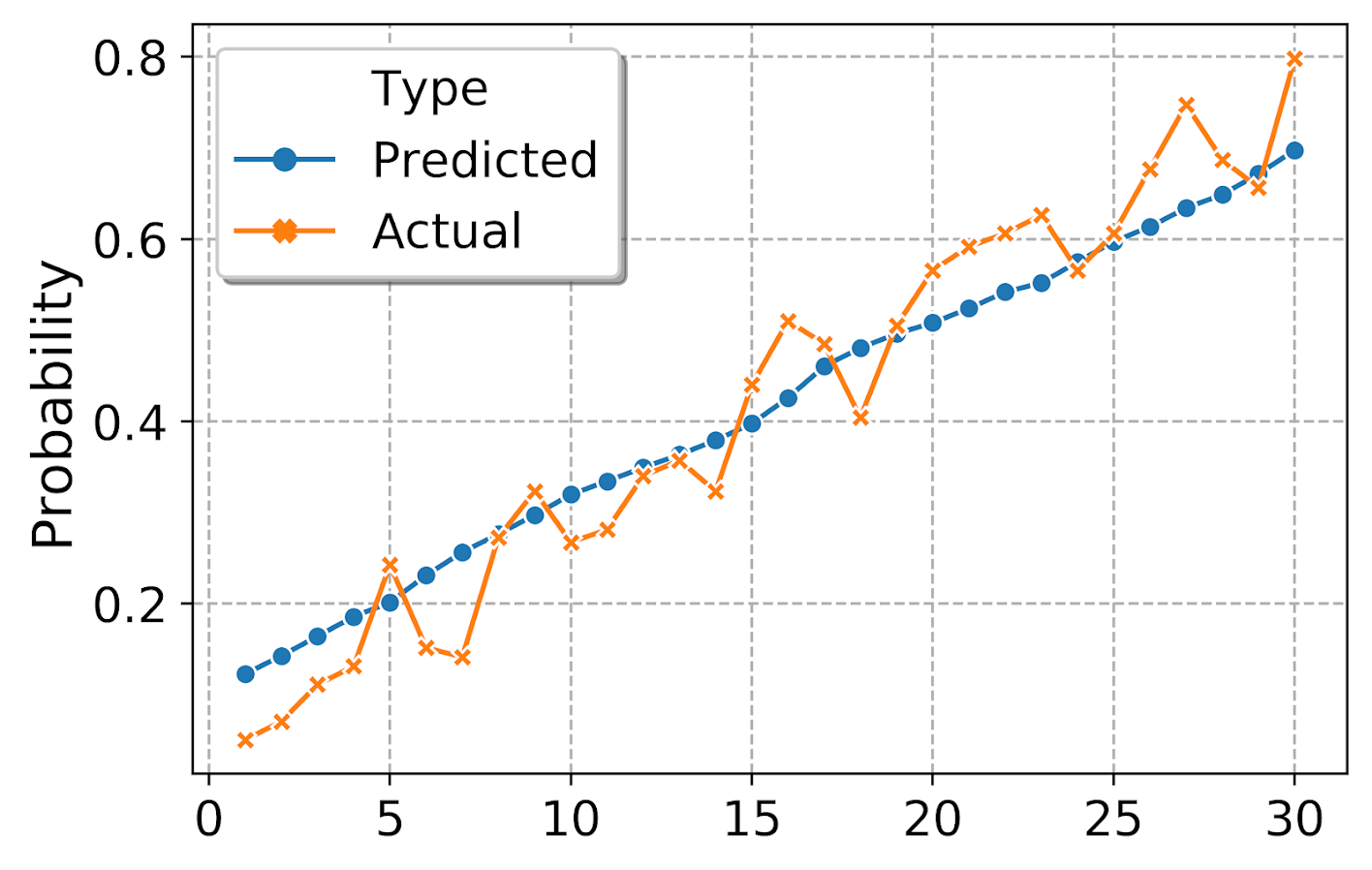By default seaborn adds in a legend title – although it is not stuffed into the actual legend title slot. (This is because they will handle multiple sub-aesthetics more gracefully I think, e.g. map color to one attribute and dash types to another.) But here I just want to get rid of it. (Similar to maps, no need to give a legend the title “legend” – should be obvious.) Also the legend did not inherit the white edge colors, so I set that as well.

#Now lets edit the legend
plt.figure(figsize=(6,4))
ax = sns.lineplot(x='Bin', y='Probability', hue='Type', style='Type', data=agg_long, dashes=False,
markers=True, markeredgecolor='w')
plt.xlabel(None)
handles, labels = ax.get_legend_handles_labels()
for i in handles:
i.set_markeredgecolor('w')
legend = ax.legend(handles=handles[1:], labels=labels[1:])
plt.savefig('sns_lift_edited_leg.png', dpi=500, bbox_inches='tight')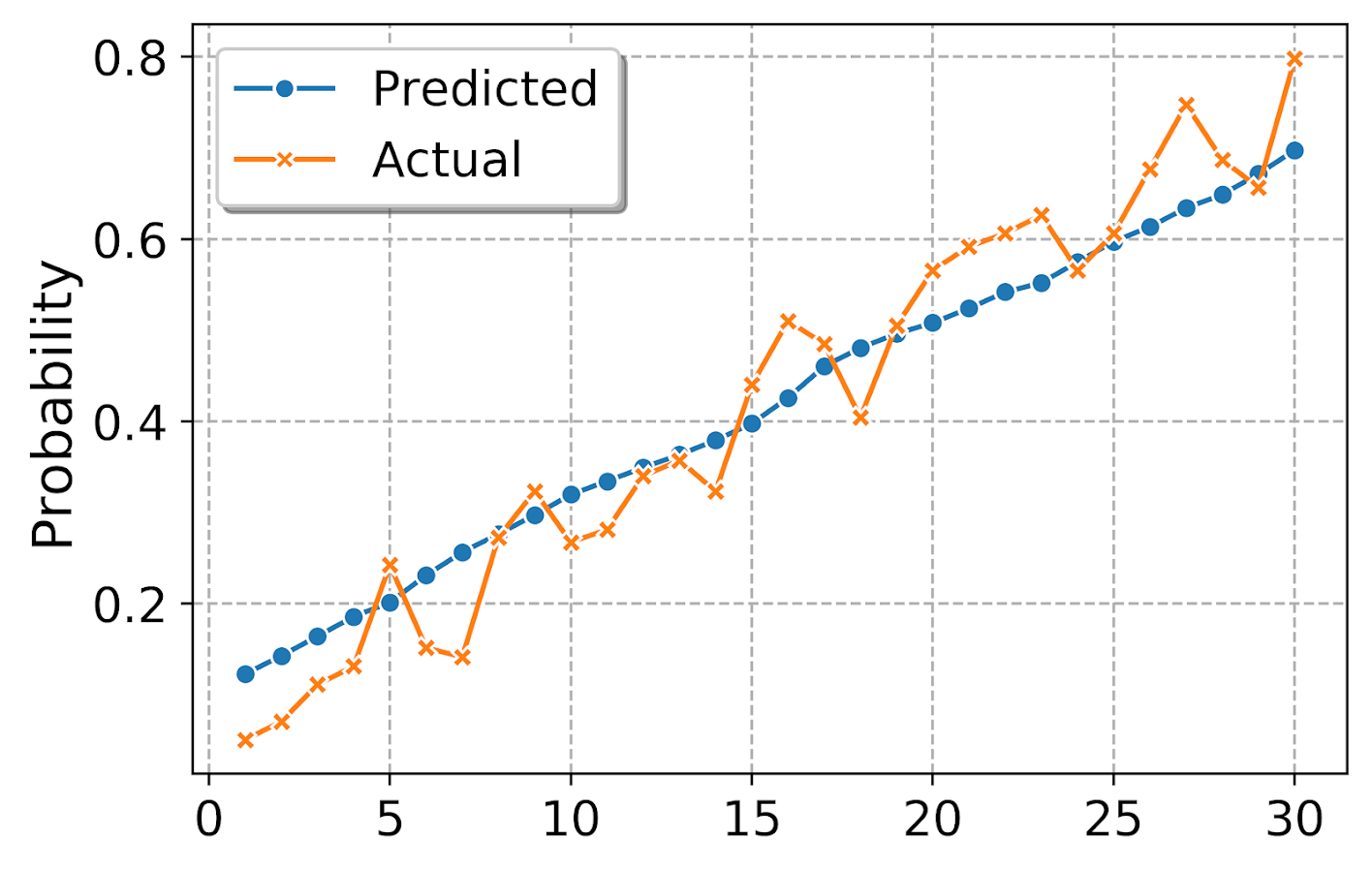# making a small multiple plot

Another nicety of seaborn is that it can make small multiple plots for you. So here I conduct analysis of calibration among subsets of data for different racial categories. First I collapse the different racial subsets into an other category, then I do the same qcut, but within the different groupings. To figure that out, I do what all good programmers do, google it and adapt from a stackoverflow example.

#########################################################
#replace everyone not black/white as other
print( recid_test['race'].value_counts() )
other_group = ['Hispanic','Other','Asian','Native American']
recid_test['RaceComb'] = recid_test['race'].replace(other_group, 'Other')
print(recid_test['RaceComb'].value_counts() )

#qcut by group
bin_sub = 20
recid_test['BinRace'] = (recid_test.groupby('RaceComb',as_index=False)['prob']
).transform( lambda x: pd.qcut(x, bin_sub, labels=range(bin_sub))
).astype(int) + 1

#Now aggregate two categories, and then melt
race_bins = recid_test.groupby(['BinRace','RaceComb'], as_index=False)['Recid30','prob','Count'].sum()
race_bins['Predicted'] = race_bins['prob']/race_bins['Count']
race_bins['Actual'] = race_bins['Recid30']/race_bins['Count']
race_long = pd.melt(race_bins, id_vars=['BinRace','RaceComb'], value_vars=['Predicted','Actual'], var_name='Type', value_name='Probability')

#Now making the small multiple plot
d = {'marker': ['o','X']}
ax = sns.FacetGrid(data=race_long, col='RaceComb', hue='Type', hue_kws=d,
col_wrap=2, despine=False, height=4)
ax.map(plt.plot, 'BinRace', 'Probability', markeredgecolor="w")
ax.set_titles("{col_name}")
ax.set_xlabels("")
#plt.legend(loc="upper left")
plt.legend(bbox_to_anchor=(1.9,0.8))
plt.savefig('sns_smallmult_niceleg.png', dpi=500, bbox_inches='tight')
#########################################################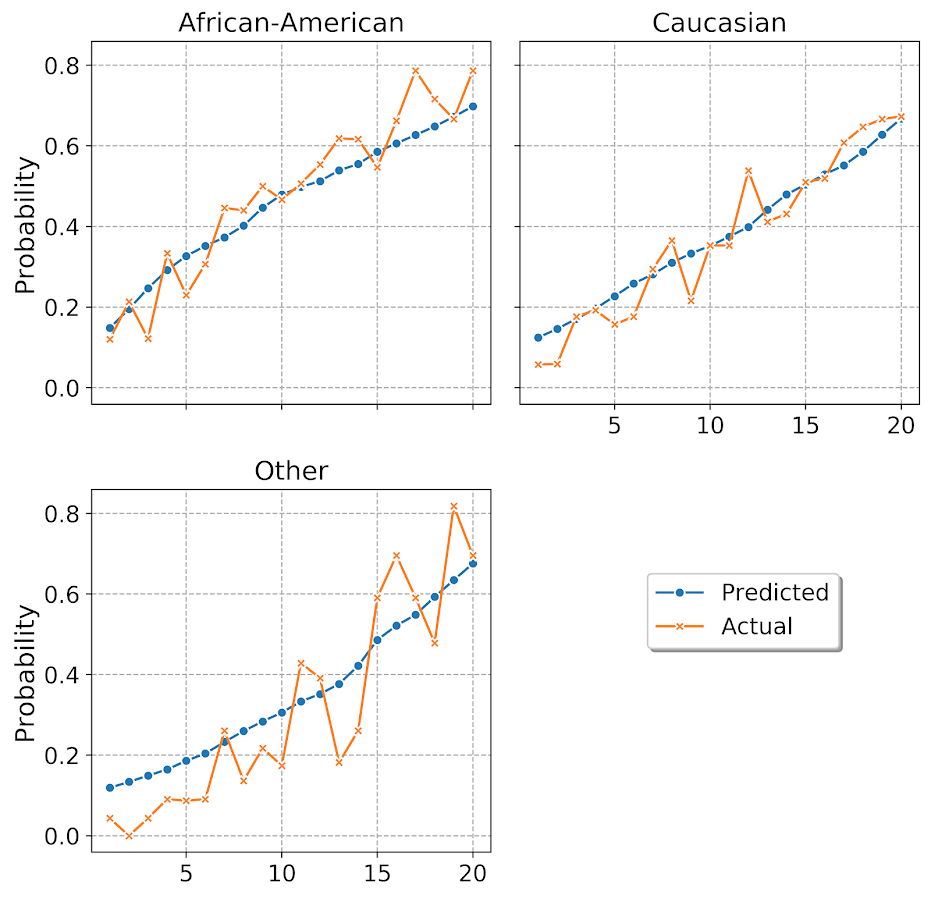And you can see that the model is fairly well calibrated for each racial subset of the data. The other category is more volatile, but it has a smaller number of observations as well. But overall does not look too bad. (If you take out my end leaf needs 100 samples though, all of these calibration plots look really bad!)

I am having a hellishly hard time doing the map of sns.lineplot to the sub-charts, but you can just do normal matplotlib plots. When you set the legend, it defaults to the last figure that was drawn, so one way to set it where you want is to use bbox_to_anchor and just test to see where it is a good spot (can use negative arguments in this function to go to the left more). Seaborn has not nice functions to map the grid text names using formatted string substitution. And the post is long enough, you can play around yourself to see how the other options change the look of the plot.

For a few notes on various gotchas I’ve encountered so far:

• For sns.FacetGrid, you need to set the size of the plots in that call, not by instantiating plt.figure(figsize=(6,4)). (This is because it draws multiple figures.)
• When drawing elements in the chart, even for named arguments the order often matters. You often need to do things like color first before other arguments for aethetics. (I believe my problem mapping sns.lineplot to my small multiple is some inheritance problems where plt.plot does not have named arguments for x/y, but sns.lineplot does.)
• To edit the legend in a FacetGrid generated set of charts, ax returns a grid, not just one element. Since each grid inherits the same legend though, you can do handles, labels = ax.get_legend_handles_labels() to get the legend handles to edit if you want.